# Common Core: High School - Geometry : Construct basic geometric figures

## Example Questions

### Example Question #1 : Construct Basic Geometric Figures

What must occur to construct two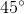angles from aangle?

Move the terminal ray of the angle up.

Move the nonterminal ray of the angle down.

Bisect thein half.

Move the terminal ray of the angle down.

Move the nonterminal ray of the angle up.

Bisect thein half.

Explanation:

To construct twoangles from aangle a bisection must occur. Recall that bisection means to cut an angle into two equal parts.

Since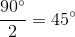that makes a bisection of theangle equal twoangles and thus is the solution.

Moving the terminal ray will not create two angles and thus it cannot be the solution.

Nonterminal is not the correct terminology and therefore, it cannot be the correct solution.

### Example Question #211 : Congruence

How are congruent lines identified in a parallelogram?

One single hash mark on one line and a double hash mark on the parallel line

Double hash marks

Either single or double hash marks

Single hash marks

Either single or double hash marks

Explanation:

Parallel lines by definition are lines that will never intersect one another. This means the slope of the lines are the same. When these lines make up a parallelogram figures that are created include squares, rectangles, diamonds, and rhombi.

Some images that depict parallelograms are as follows. Notice that either single or double hash marks can be used to identify that opposite lines are congruent.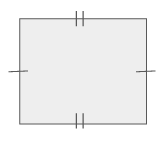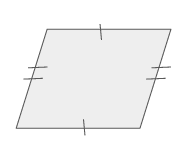### Example Question #211 : Congruence

Given the point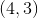, how would perpendicular lines be constructed?

Perpendicular lines are done by drawing a vertical line at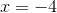and a horizontal line at.

Perpendicular lines are done by drawing a vertical line at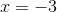and a horizontal line at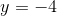.

Perpendicular lines are done by drawing a vertical line atand a horizontal line at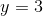.

Perpendicular lines are done by drawing a vertical line atand a horizontal line at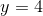.

Perpendicular lines are done by drawing a vertical line atand a horizontal line at.

Perpendicular lines are done by drawing a vertical line atand a horizontal line at.

Explanation:

To construct perpendicular lines first recall what it means to be perpendicular.

Recall that perpendicular lines intersect at one point and have opposite, reciprocal slopes.

Plotting the given point is as follows: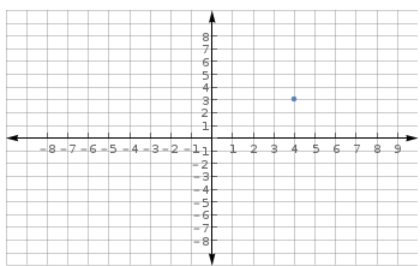Given this point, there are numerous combinations of perpendicular lines that can be made. Looking at the possible options the only pair that intersect at the pointis when a vertical line is drawn atand a horizontal line at.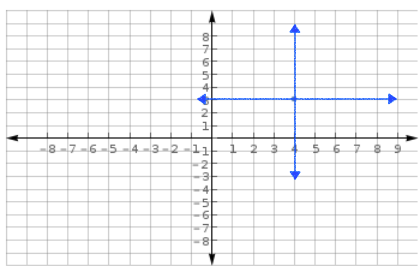### Example Question #581 : High School: Geometry

Determine whether the statement is true or false:

Lines that have fractional slopes cannot be perpendicular to other lines.

True

False

False

Explanation:

For two lines to be perpendicular they must intersect at one point and have the opposite reciprocals on each other. Having slopes that are opposite reciprocals creates aangle between the two lines which is also by definition makes the two lines perpendicular.

Therefore, a line that has a fractional slope can also have a perpendicular line. If the line in question has a slope of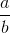then the perpendicular line would have a slope of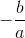.

Thus, the statement, "Lines that have fractional slopes cannot be perpendicular to other lines." is false.

### Example Question #212 : Congruence

Determine whether the statement is true or false:

An equilateral triangle must have sides of equal length but the interior angles may differ.

False

True

False

Explanation:

By definition an equilateral triangle has all sides that are of equal length and angles are also equal. All interior angles on an equilateral triangle equalregardless of the side lengths.

Therefore, the statement "An equilateral triangle must have sides of equal length but the interior angles may differ." is false.

### Example Question #1 : Construct Basic Geometric Figures

Determine whether the statement is true or false:

In a parallelogram, opposite sides are always congruent.

False

True